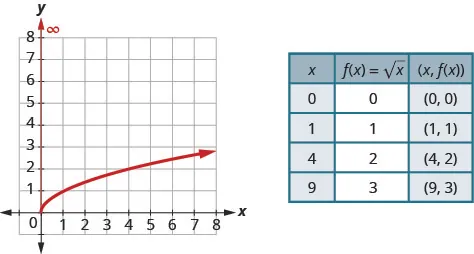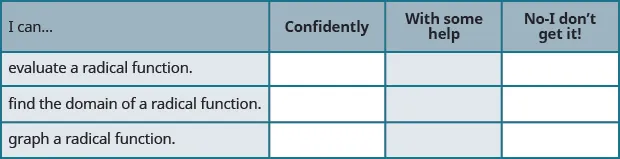Intermediate Algebra

Intermediate Algebra8.7 Use Radicals in Functions

### Learning Objectives

By the end of this section, you will be able to:
• Find the domain of a radical function

### Be Prepared 8.7

Before you get started, take this readiness quiz.

1. Solve: $1−2x≥0.1−2x≥0.$
If you missed this problem, review Example 2.50.
2. For $f(x)=3x−4,f(x)=3x−4,$ evaluate $f(2),f(−1),f(0).f(2),f(−1),f(0).$
If you missed this problem, review Example 3.48.
3. Graph $f(x)=x.f(x)=x.$ State the domain and range of the function in interval notation.
If you missed this problem, review Example 3.56.

In this section we will extend our previous work with functions to include radicals. If a function is defined by a radical expression, we call it a radical function.

The square root function is $f(x)=x.f(x)=x.$

The cube root function is $f(x)=x3.f(x)=x3.$

A radical function is a function that is defined by a radical expression.

To evaluate a radical function, we find the value of f(x) for a given value of x just as we did in our previous work with functions.

### Example 8.68

For the function $f(x)=2x−1,f(x)=2x−1,$ find $f(5)f(5)$ $f(−2).f(−2).$

### Try It 8.135

For the function $f(x)=3x−2,f(x)=3x−2,$ find $f(6)f(6)$ $f(0).f(0).$

### Try It 8.136

For the function $g(x)=5x+5,g(x)=5x+5,$ find $g(4)g(4)$ $g(−3).g(−3).$

We follow the same procedure to evaluate cube roots.

### Example 8.69

For the function $g(x)=x−63,g(x)=x−63,$ find $g(14)g(14)$ $g(−2).g(−2).$

### Try It 8.137

For the function $g(x)=3x−43,g(x)=3x−43,$ find $g(4)g(4)$ $g(1).g(1).$

### Try It 8.138

For the function $h(x)=5x−23,h(x)=5x−23,$ find $h(2)h(2)$ $h(−5).h(−5).$

The next example has fourth roots.

### Example 8.70

For the function $f(x)=5x−44,f(x)=5x−44,$ find $f(4)f(4)$ $f(−12)f(−12)$

### Try It 8.139

For the function $f(x)=3x+44,f(x)=3x+44,$ find $f(4)f(4)$ $f(−1).f(−1).$

### Try It 8.140

For the function $g(x)=5x+14,g(x)=5x+14,$ find $g(16)g(16)$ $g(3).g(3).$

### Find the Domain of a Radical Function

To find the domain and range of radical functions, we use our properties of radicals. For a radical with an even index, we said the radicand had to be greater than or equal to zero as even roots of negative numbers are not real numbers. For an odd index, the radicand can be any real number. We restate the properties here for reference.

### Properties of $a n a n$

When n is an even number and:

• $a≥0,a≥0,$ then $anan$ is a real number.
• $a<0,a<0,$ then $anan$ is not a real number.

When n is an odd number, $anan$ is a real number for all values of a.

So, to find the domain of a radical function with even index, we set the radicand to be greater than or equal to zero. For an odd index radical, the radicand can be any real number.

### Domain of a Radical Function

When the index of the radical is even, the radicand must be greater than or equal to zero.

When the index of the radical is odd, the radicand can be any real number.

### Example 8.71

Find the domain of the function, $f(x)=3x−4.f(x)=3x−4.$ Write the domain in interval notation.

### Try It 8.141

Find the domain of the function, $f(x)=6x−5.f(x)=6x−5.$ Write the domain in interval notation.

### Try It 8.142

Find the domain of the function, $f(x)=4−5x.f(x)=4−5x.$ Write the domain in interval notation.

### Example 8.72

Find the domain of the function, $g(x)=6x−1.g(x)=6x−1.$ Write the domain in interval notation.

### Try It 8.143

Find the domain of the function, $f(x)=4x+3.f(x)=4x+3.$ Write the domain in interval notation.

### Try It 8.144

Find the domain of the function, $h(x)=9x−5.h(x)=9x−5.$ Write the domain in interval notation.

The next example involves a cube root and so will require different thinking.

### Example 8.73

Find the domain of the function, $f(x)=2x2+33.f(x)=2x2+33.$ Write the domain in interval notation.

### Try It 8.145

Find the domain of the function, $f(x)=3x2−13.f(x)=3x2−13.$ Write the domain in interval notation.

### Try It 8.146

Find the domain of the function, $g(x)=5x−43.g(x)=5x−43.$ Write the domain in interval notation.

Before we graph any radical function, we first find the domain of the function. For the function, $f(x)=x,f(x)=x,$ the index is even, and so the radicand must be greater than or equal to 0.

This tells us the domain is $x≥0x≥0$ and we write this in interval notation as $[0,∞).[0,∞).$

Previously we used point plotting to graph the function, $f(x)=x.f(x)=x.$ We chose x-values, substituted them in and then created a chart. Notice we chose points that are perfect squares in order to make taking the square root easier.Once we see the graph, we can find the range of the function. The y-values of the function are greater than or equal to zero. The range then is $[0,∞).[0,∞).$

### Example 8.74

For the function $f(x)=x+3,f(x)=x+3,$

find the domain graph the function use the graph to determine the range.

### Try It 8.147

For the function $f(x)=x+2,f(x)=x+2,$ find the domain graph the function use the graph to determine the range.

### Try It 8.148

For the function $f(x)=x−2,f(x)=x−2,$ find the domain graph the function use the graph to determine the range.

In our previous work graphing functions, we graphed $f(x)=x3f(x)=x3$ but we did not graph the function $f(x)=x3.f(x)=x3.$ We will do this now in the next example.

### Example 8.75

For the function $f(x)=x3,f(x)=x3,$ find the domain graph the function use the graph to determine the range.

### Try It 8.149

For the function $f(x)=−x3,f(x)=−x3,$

find the domain graph the function use the graph to determine the range.

### Try It 8.150

For the function $f(x)=x−23,f(x)=x−23,$

find the domain graph the function use the graph to determine the range.

### Media

Access these online resources for additional instruction and practice with radical functions.

### Section 8.7 Exercises

#### Practice Makes Perfect

In the following exercises, evaluate each function.

351.

$f(x)=4x−4,f(x)=4x−4,$ find $f(5)f(5)$ $f(0).f(0).$

352.

$f(x)=6x−5,f(x)=6x−5,$ find $f(5)f(5)$ $f(−1).f(−1).$

353.

$g(x)=6x+1,g(x)=6x+1,$ find $g(4)g(4)$ $g(8).g(8).$

354.

$g(x)=3x+1,g(x)=3x+1,$ find $g(8)g(8)$ $g(5).g(5).$

355.

$F(x)=3−2x,F(x)=3−2x,$ find $F(1)F(1)$ $F(−11).F(−11).$

356.

$F(x)=8−4x,F(x)=8−4x,$ find $F(1)F(1)$ $F(−2).F(−2).$

357.

$G(x)=5x−1,G(x)=5x−1,$ find $G(5)G(5)$ $G(2).G(2).$

358.

$G(x)=4x+1,G(x)=4x+1,$ find $G(11)G(11)$ $G(2).G(2).$

359.

$g(x)=2x−43,g(x)=2x−43,$ find $g(6)g(6)$ $g(−2).g(−2).$

360.

$g(x)=7x−13,g(x)=7x−13,$ find $g(4)g(4)$ $g(−1).g(−1).$

361.

$h(x)=x2−43,h(x)=x2−43,$ find $h(−2)h(−2)$ $h(6).h(6).$

362.

$h(x)=x2+43,h(x)=x2+43,$ find $h(−2)h(−2)$ $h(6).h(6).$

363.

For the function $f(x)=2x34,f(x)=2x34,$ find $f(0)f(0)$ $f(2).f(2).$

364.

For the function $f(x)=3x34,f(x)=3x34,$ find $f(0)f(0)$ $f(3).f(3).$

365.

For the function $g(x)=4−4x4,g(x)=4−4x4,$ find $g(1)g(1)$ $g(−3).g(−3).$

366.

For the function $g(x)=8−4x4,g(x)=8−4x4,$ find $g(−6)g(−6)$ $g(2).g(2).$

Find the Domain of a Radical Function

In the following exercises, find the domain of the function and write the domain in interval notation.

367.

$f ( x ) = 3 x − 1 f ( x ) = 3 x − 1$

368.

$f ( x ) = 4 x − 2 f ( x ) = 4 x − 2$

369.

$g ( x ) = 2 − 3 x g ( x ) = 2 − 3 x$

370.

$g ( x ) = 8 − x g ( x ) = 8 − x$

371.

$h ( x ) = 5 x − 2 h ( x ) = 5 x − 2$

372.

$h ( x ) = 6 x + 3 h ( x ) = 6 x + 3$

373.

$f ( x ) = x + 3 x − 2 f ( x ) = x + 3 x − 2$

374.

$f ( x ) = x − 1 x + 4 f ( x ) = x − 1 x + 4$

375.

$g ( x ) = 8 x − 1 3 g ( x ) = 8 x − 1 3$

376.

$g ( x ) = 6 x + 5 3 g ( x ) = 6 x + 5 3$

377.

$f ( x ) = 4 x 2 − 16 3 f ( x ) = 4 x 2 − 16 3$

378.

$f ( x ) = 6 x 2 − 25 3 f ( x ) = 6 x 2 − 25 3$

379.

$F ( x ) = 8 x + 3 4 F ( x ) = 8 x + 3 4$

380.

$F ( x ) = 10 − 7 x 4 F ( x ) = 10 − 7 x 4$

381.

$G ( x ) = 2 x − 1 5 G ( x ) = 2 x − 1 5$

382.

$G ( x ) = 6 x − 3 5 G ( x ) = 6 x − 3 5$

In the following exercises, find the domain of the function graph the function use the graph to determine the range.

383.

$f ( x ) = x + 1 f ( x ) = x + 1$

384.

$f ( x ) = x − 1 f ( x ) = x − 1$

385.

$g ( x ) = x + 4 g ( x ) = x + 4$

386.

$g ( x ) = x − 4 g ( x ) = x − 4$

387.

$f ( x ) = x + 2 f ( x ) = x + 2$

388.

$f ( x ) = x − 2 f ( x ) = x − 2$

389.

$g ( x ) = 2 x g ( x ) = 2 x$

390.

$g ( x ) = 3 x g ( x ) = 3 x$

391.

$f ( x ) = 3 − x f ( x ) = 3 − x$

392.

$f ( x ) = 4 − x f ( x ) = 4 − x$

393.

$g ( x ) = − x g ( x ) = − x$

394.

$g ( x ) = − x + 1 g ( x ) = − x + 1$

395.

$f ( x ) = x + 1 3 f ( x ) = x + 1 3$

396.

$f ( x ) = x − 1 3 f ( x ) = x − 1 3$

397.

$g ( x ) = x + 2 3 g ( x ) = x + 2 3$

398.

$g ( x ) = x − 2 3 g ( x ) = x − 2 3$

399.

$f ( x ) = x 3 + 3 f ( x ) = x 3 + 3$

400.

$f ( x ) = x 3 − 3 f ( x ) = x 3 − 3$

401.

$g ( x ) = x 3 g ( x ) = x 3$

402.

$g ( x ) = − x 3 g ( x ) = − x 3$

403.

$f ( x ) = 2 x 3 f ( x ) = 2 x 3$

404.

$f ( x ) = −2 x 3 f ( x ) = −2 x 3$

#### Writing Exercises

405.

Explain how to find the domain of a fourth root function.

406.

Explain how to find the domain of a fifth root function.

407.

Explain why $y=x3y=x3$ is a function.

408.

Explain why the process of finding the domain of a radical function with an even index is different from the process when the index is odd.

#### Self Check

After completing the exercises, use this checklist to evaluate your mastery of the objectives of this section.What does this checklist tell you about your mastery of this section? What steps will you take to improve?

Order a print copy

As an Amazon Associate we earn from qualifying purchases.